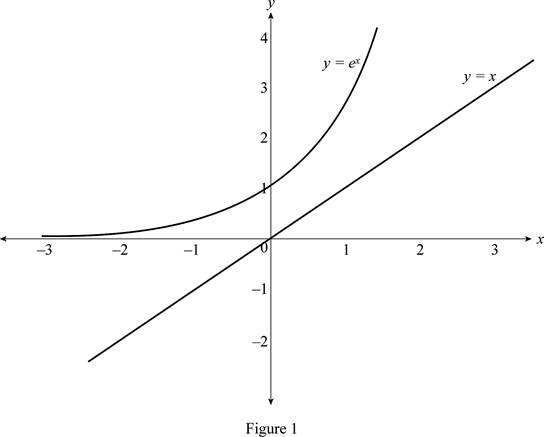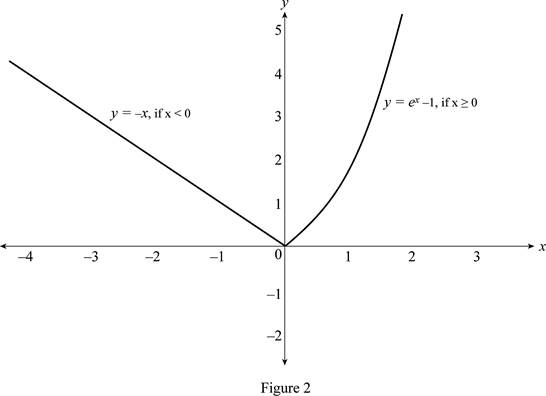# The graph of the function f ( x ) = { − x if x &lt; 0 e x − 1 if x ≥ 0 by using transformation.### Single Variable Calculus: Concepts...

4th Edition
James Stewart
Publisher: Cengage Learning
ISBN: 9781337687805### Single Variable Calculus: Concepts...

4th Edition
James Stewart
Publisher: Cengage Learning
ISBN: 9781337687805

#### Solutions

Chapter 1, Problem 16RE
To determine

## To sketch: The graph of the function f(x)={−x      if x<0ex−1  if x≥0 by using transformation.

Expert Solution

### Explanation of Solution

Since the given function is the step function, there are two functions such as, y=x,if x<0 and y=ex1,if x0.

The standard graph of the function y=ex and y=x is roughly drawn and shown below in Figure 1.Then, to draw the graph of y=ex1,if x0, shift the graph y=ex one unit downward and consider only the first quadrant portion .

Similarly, to draw the of y=x,if x<0, reflect the graph y=x about y-axis and consider the only the second quadrant part.

Thus, the graph of f(x)={x      if x<0ex1  if x0 is shown below in Figure 2.From figure 2, it is observed that the graph y=ex1 is drawn for the numbers x0 and the graph y=x is drawn for the numbers x<0.

### Have a homework question?

Subscribe to bartleby learn! Ask subject matter experts 30 homework questions each month. Plus, you’ll have access to millions of step-by-step textbook answers!